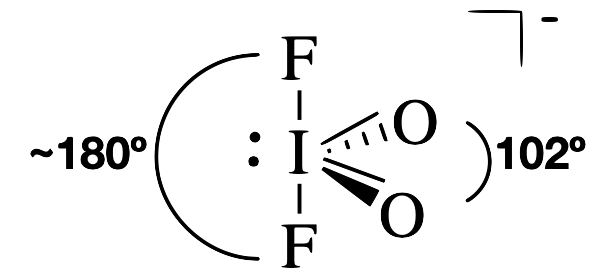# 1.3.2.2: Multiple Bonds

•• Kathryn Haas
• Duke University
$$\newcommand{\vecs}{\overset { \rightharpoonup} {\mathbf{#1}} }$$ $$\newcommand{\vecd}{\overset{-\!-\!\rightharpoonup}{\vphantom{a}\smash {#1}}}$$$$\newcommand{\id}{\mathrm{id}}$$ $$\newcommand{\Span}{\mathrm{span}}$$ $$\newcommand{\kernel}{\mathrm{null}\,}$$ $$\newcommand{\range}{\mathrm{range}\,}$$ $$\newcommand{\RealPart}{\mathrm{Re}}$$ $$\newcommand{\ImaginaryPart}{\mathrm{Im}}$$ $$\newcommand{\Argument}{\mathrm{Arg}}$$ $$\newcommand{\norm}{\| #1 \|}$$ $$\newcommand{\inner}{\langle #1, #2 \rangle}$$ $$\newcommand{\Span}{\mathrm{span}}$$ $$\newcommand{\id}{\mathrm{id}}$$ $$\newcommand{\Span}{\mathrm{span}}$$ $$\newcommand{\kernel}{\mathrm{null}\,}$$ $$\newcommand{\range}{\mathrm{range}\,}$$ $$\newcommand{\RealPart}{\mathrm{Re}}$$ $$\newcommand{\ImaginaryPart}{\mathrm{Im}}$$ $$\newcommand{\Argument}{\mathrm{Arg}}$$ $$\newcommand{\norm}{\| #1 \|}$$ $$\newcommand{\inner}{\langle #1, #2 \rangle}$$ $$\newcommand{\Span}{\mathrm{span}}$$

In the previous sections, we saw how to predict the approximate geometry around an atom using VSEPR theory, and we learned that lone pairs of electrons slightly distort bond angles from the "parent" geometry. This page discusses the effect of multiple (double and triple) bonds between bonded atoms.

## Double and triple bonds are more repulsive than single bonds

VSEPR theory predicts that double and triple bonds have stronger repulsive forces than single bonds. Like lone pairs of electrons, multiple bonds occupy more space around the central atom than a single bond. The result is that bond angles are slightly distorted compared to the parent geometry. Since a multiple bond has a higher electron density than a single bond, its electrons occupy more space than those of a single bond. Double and triple bonds distort bond angles in a similar way as do lone pairs. Due to the stronger repulsion, double and triple bonds occupy similar positions as lone pairs in groups with 5 and 6 electron groups.

#### CH2O

In a molecule such as CH2O (AX3), whose structure is shown below, the double bond repels the single bonds more strongly than the single bonds repel each other. This causes a deviation from ideal geometry (an H–C–H bond angle of 116.5° rather than 120°).#### 2-methybutene

In the molecule, CH2C(CH3)2, the methyl—C—methyl bond angle is 115.6°, which is less than the 120° bond angle that would be expected of the parent geometry. On the other hand, the acetyl—C—methyl bond angle is greater than 120°, with an actual bond angle of 122.2°.## Atoms with both lone pairs and multiple bonds

In general, we expect that a lone pair has slightly greater repulsive force than a multiple bond, and that a multiple bond has slightly greater repulsive force than a single bond. The order of expected repulsive force is:

$\text{lone pair} > \text{double or triple bond} > \text{single bond}$

#### IO2F2¯

With five electron groups, the IO2F2 molecule has an approximately trigonal bipyramidal electronic (parent) geometry. The central iodine atom has a single bond to each of the fluorine atoms, a double bond to each oxygen, and a lone pair. The lone pair and the double bonds will occupy more space around iodine than each of the single bonds. Thus, we expect the lone pair and double bonds to occupy equatorial positions around the central iodine. The O=I=O bond angle is 102° (much smaller than the 120° angle expected from the parent geometry). This dramatically smaller angle is a result of the increased repulsion between the lone pair and the double bond.#### SeOCl2

With four electron groups, the SeOCl2 molecule has four electron grouns and approximately tetrahedral electronic (parent) geometry. It has both a lone pair and a double bond on the central selenium atom. The two chlorine atoms are singly bonded to selenium, while the oxygen is double bonded. The lone pair is most repulsive, followed by the double bonded oxygen, and then the chlorine bonds. This gives a Cl—Se—Cl bond angle of 97° and a Cl—Se—O bond angle of 106°; both angles are less than the 109.5° angles expected for the ideal tetrahedral geometry.## Practice

Exercise $$\PageIndex{1}$$

Use VSEPR theory to predict the geometries and draw the structures of the following.

1. XeOF4
2. NO2¯
3. SOCl2
4. IOF3¯

This molecule has six electron groups around the central Xe atom (steric number 6), and thus has an approximately octahdral electronic (parent) geometry. There is a double bond to O and a lone pair that are more strongly repulsive than the single bonds to F. The double bond and lone pair will be directly opposite of each other, designated as axial position. The result is a square pyramid molecular geometry. VSEPR theory predicts F—Xe—F bond angles of 90°. As a general rule, lone pairs are slightly more repulsive than multiple bonds, and so we might expect the O—Xe—F bond angles to be <90°; however in this case the actual O—Xe—F bond angle is observed to be 91° (you could not have predicted the latter).This molecule has three electron groups around the central atom: one lone pair and two double bonds to oxygen atoms. This results in an approximately trigonal planar electronic (parent geometry). We expect the lone pair to be slightly more repulsive than double bonds, and so we expect the O—N—O bond angle to be slightly less than 120°. The actual O—N—O angle is 115°.This molecule has four electron groups (steric number 4) with an approximately tetrahedral electronic (parent) geometry. Each double bonded oxygen will take up more space around the central S than the single bonded Cl atoms. We should expect the double bonds to repel each other more strongly than they repel each single bond. With the least repulsive interaction being between the two single bonds. The bond angle for Cl—S—Cl is expected to be <109.5° according to VSEPR theory (however it is actually 111°). We would expect the bond angle for O—S—O to be larger than the angle for Cl—S—Cl and for Cl—S—O. The O—S—O bond angle is predicted to be >109.5° (and the actual bond angle is 120°). The molecular geometry is a distorted tetrahedron.